## Which expression represents the distance between the points (11, 4) and (5,8)?

Question

Which expression represents the distance between the points (11, 4) and (5,8)?

in progress 0
4 weeks 2021-08-17T12:20:24+00:00 1 Answers 2 views 0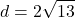General Formulas and Concepts:

Pre-Algebra

Order of Operations: BPEMDAS

1. Brackets
2. Parenthesis
3. Exponents
4. Multiplication
5. Division
7. Subtraction
• Left to Right

Algebra I

• Coordinates (x, y)

Algebra II

• Distance Formula: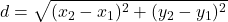Step-by-step explanation:

Step 1: Define

Point (11, 4) → x₁ = 11, y₁ = 4

Point (5, 8) → x₂ = 5, y₂ = 8

Step 2: Find distance d

Simply plug in the 2 coordinates into the distance formula to find distance d

1. Substitute in points [Distance Formula]: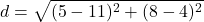2. [√Radical] (Parenthesis) Subtract: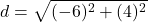3. [√Radical] Evaluate exponents: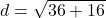4. [√Radical] Add: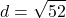5. [√Radical] Simplify: